# How to multiply polynomials

We write a rule of multiplication of polynomials and consider how to multiply polynomials on specific examples.

The rule of multiplication of polynomials.

To multiply a polynomial to the polynomial, each member of one polynomials multiply to each member of the other polynomial and the resulting works are folded.

With the help of letters multiplication of polynomials (in this case, it is three-metered on biccoon) can be written as: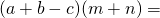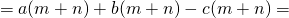At the initial stage of studying the topic, it makes sense to be several very first examples in the same detail.

For example,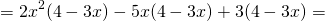Now we multiply the same on the polynomial and give such members: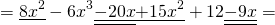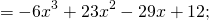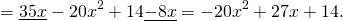How to learn to multiply polynomials without a detailed record?

First we imagine that the first term of the first brackets we multiply on the polynomial in the second brackets, then the second term from the first brackets to multiply on the second brackets, and so on. You can even help yourself, closing the rest of the aligned handle or pencil.

For example,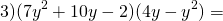Multiply the first term of the first brackets, 7Y², for each term from the second brackets. Before 7Y² there is a sign "+", then the second brackets costs "+". So, signs in brackets do not change. We get 28Y³-7y.

Then we multiply the second term from the first brackets, 10y, for each term from the second brackets. Before 10Y there is a sign "+", signs in brackets do not change: 40y²-10y³.

Go to the multiplication of the third term of the first brackets, -2, for each sample of the second brackets. Before -2 there is a sign "-", each sign in brackets change to the opposite: -8Y + 2Y².

All together are written so:Now we give similar terms: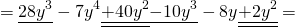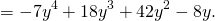A little practice, and you make sure to multiply polynomials is not difficult. Only the attentiveness and knowledge of the previous mother is important.